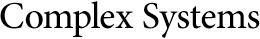## Cyclic Evolution of Neuronal Automata with Memory When All the Weighting Coefficients are Strictly Positive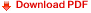René Ndoundam
Département d'Informatique,
Faculté des Sciences,
Université de Yaoundé I,
B.P 812 Yaoundé, Cameroun

Martín Matamala
In this paper we study the sequences generated by a neuronal equation of the form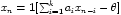, where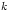is the size of the memory. We show that in the case where all the parameters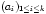are strictly positive reals andis a multiple of 6 (i.e.,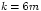), there exists a subset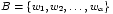of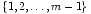and for which there exists a neuronal equation of memory length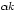(i.e.,) with strictly positive weighting coefficients that generates a sequence of period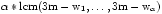. As an application; first, for all integer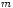greater than or equal to 3, we exhibit a neuronal recurrence equation of memory length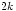with weighting coefficients that are all strictly positive and which generates a sequence of period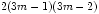; and second, for all odd integergreater than or equal to 5, we exhibit a neuronal equation of memory length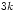with strictly positive weighting coefficients that generates a sequence of period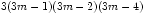.